# Direction of current flow?

In the following diagrams: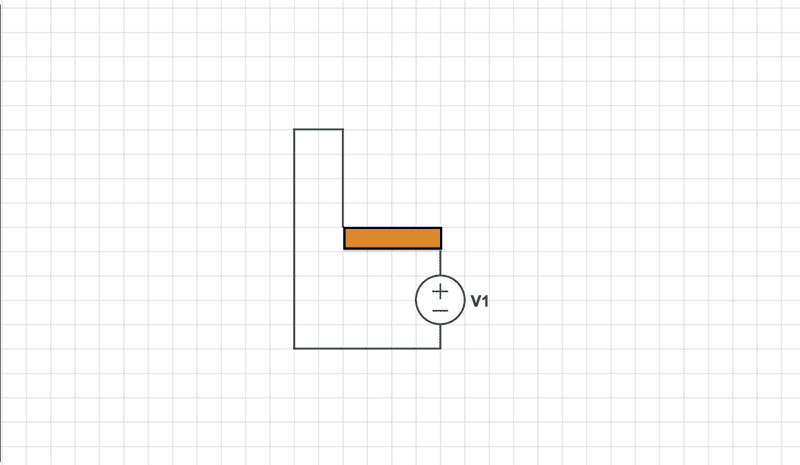and: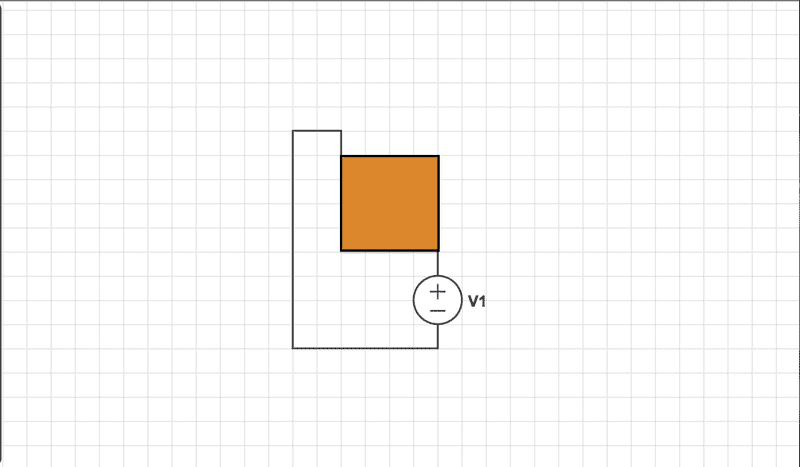Is current flowing horizontal or vertical&horizontal?
I'm sure for the rectangle one the flow of current is horizontal, but not sure of that square.

dlgoff
Gold Member
This "analogy"(?) doesn't tell me anything about current. If anything, it might suggest a higher potential in the second one.

donpacino
Gold Member
In both of the diagrams the current flow is both vertical and horizontal.
Assuming the voltage source has a positive value, current will flow from the voltage source into the bottom right corner of the rectangle and then to the upper left corner of the rectangle, then back around to the source.

That being said, asking if the current flow is vertical or horizontal is a very strange question.

Why do you need to know this?

This "analogy"(?) doesn't tell me anything about current. If anything, it might suggest a higher potential in the second one.

Why? It's quite simple, a voltage source connected to different shapes of conductors.
I wanted to know if they flow of current would be strictly horizontal on the first one, and both horizontal and vertical for the second.

In both of the diagrams the current flow is both vertical and horizontal.
Assuming the voltage source has a positive value, current will flow from the voltage source into the bottom right corner of the rectangle and then to the upper left corner of the rectangle, then back around to the source.

To be exact, can the direction of current be assumed like so: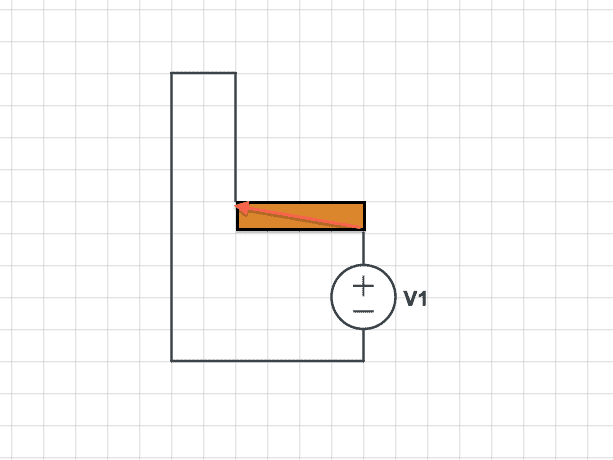And: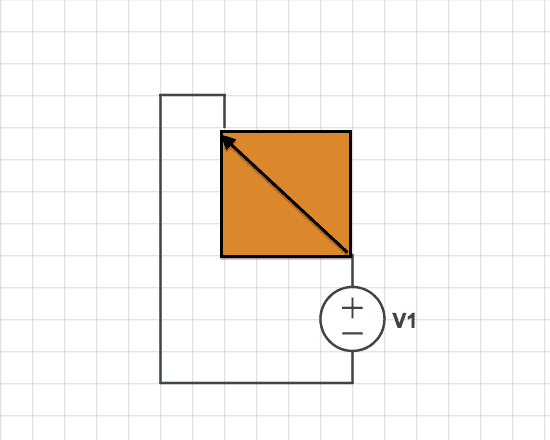Is it evenly divided, as to how some current flow vertical and some current flows horizontal(50/50)?

Why do you need to know this?

Wanted to see how the "shape" or dimensions of a conductor, and where it's connected had an effect on direction of current.

davenn
Gold Member
2021 Award
the current will flow throughout the conductor, not just in a narrow line across it

NascentOxygen
Staff Emeritus
If that is a conductive medium of uniform thickness, then the current spreads out in a wide swayth, it doesn't confine its path to a thin middle strip. Think of the block as many resistances all in parallel; current goes through each, though proportionally less through the higher resistance (i.e., longer) paths.
For DC, picture a block of metal as myriad resistances in 3D. http://thumbnails111.imagebam.com/37333/4d0696373324852.jpg [Broken]

Last edited by a moderator:
•PhiowPhi and davenn
davenn
Gold Member
2021 Award
thanks for the backup :)

jim hardy
Gold Member
Dearly Missed
It's like fluid flow. There'll be equipotential lines , segments of a circle centered on corners, with flow largely perpendicular
I guess that's why in my EE curriculum we had to take a course in Fluid Flow.analysis. Back in those days(slide rules) we worked them graphically like finite elements.
Only years later did i appreciate the value of that course.

•PhiowPhi
the current will flow throughout the conductor, not just in a narrow line across it

If that is a conductive medium of uniform thickness, then the current spreads out in a wide swayth, it doesn't confine its path to a thin middle strip. Think of the block as many resistances all in parallel; current goes through each, though proportionally less through the higher resistance (i.e., longer) paths.

Very interesting.

If one was to assume a uniform direction there shouldn't be one right? For such conductors? Current flow will be in all directions?
I was relating such a design to applications where current has a distinguished impact. Such as the Lorentz force, if it's true that current moves around technically everywhere, there shouldn't be a unified direction. In comparison to the examples where current flow is uniformly obvious?

Initially, it felt intuitive to assume current flow was all horizontal and some parts we're vertical(around the edges), but if it goes all around... then there shouldn't be a direction for it?
It makes sense when I think of current being a scalar quantity, but when I apply some calculations, I always mistake it for a vector.

dlgoff
Gold Member
Why? It's quite simple, a voltage source connected to different shapes of conductors.
You didn't mention conductors in your OP. Just sayin'

You didn't mention conductors in your OP. Just sayin'

Whoops! Need to go into details next time!

sophiecentaur
Gold Member
Whoops! Need to go into details next time!
You certainly do - and more precision! I was initially working on the assumption that there were connections along two edges (but which ones?). It would have been much better to have no lines round the edges and a single 'blob' at the corners where the connection is made. Your drawing package will be able to do this for you.
PF is an untamed beast and will go haring off in all sorts of directions if you don't tell it exactly what to do. Then it's a helpful pussycat.

•PhiowPhi
PF is an untamed beast and will go haring off in all sorts of directions if you don't tell it exactly what to do. Then it's a helpful pussycat.
. This... the true guidance!

Hm, I could adjust it now... but I think most of the members who posted get the idea for now.

But certainly will do in the future!

Why is it a predicament to me? Well, since current is somewhat all around the conductor(Literally...maybe?), does that mean there isn't a unified direction?
Specifically in relation to Lorentz force, how can it exist if current moving all around the wire?

donpacino
Gold Member
. This... the true guidance!

Hm, I could adjust it now... but I think most of the members who posted get the idea for now.

But certainly will do in the future!

Why is it a predicament to me? Well, since current is somewhat all around the conductor(Literally...maybe?), does that mean there isn't a unified direction?
Specifically in relation to Lorentz force, how can it exist if current moving all around the wire?

While the current does flow throughout all of the conductor, it will still flow from high to low voltage.
Therefore the general flow of current will be from the bottom right to the upper left.

•PhiowPhi
sophiecentaur
Gold Member
You can also say that the equipotential lines, right next to the connectionswill be arcs of a circle. If the area is a square, (easy bit of symmetry) there will be a diagonal equipotential line across the 'other' diagonal. In the region of that diagonal, the lines will be spaced out more (lower voltage drop because of the lower effective parallel resistance) and tighter together near the connections. The shapes of the lines will morph as you move across the square.
I just can't remember the book work to do the analysis but the arm waving picture will look as I describe.
Oh yes - and the current will always flow at right angles to the equipotential lines.

•jim hardy
While the current does flow throughout all of the conductor, it will still flow from high to low voltage.
Therefore the general flow of current will be from the bottom right to the upper left.

Great, that makes sense.

You can also say that the equipotential lines, right next to the connectionswill be arcs of a circle. If the area is a square, (easy bit of symmetry) there will be a diagonal equipotential line across the 'other' diagonal. In the region of that diagonal, the lines will be spaced out more (lower voltage drop because of the lower effective parallel resistance) and tighter together near the connections. The shapes of the lines will morph as you move across the square.
I just can't remember the book work to do the analysis but the arm waving picture will look as I describe.
Oh yes - and the current will always flow at right angles to the equipotential lines.

The wording makes sense, yet imaging it is a bit difficult, but eventually I will.

sophiecentaur
Gold Member
Great, that makes sense.

The wording makes sense, yet imaging it is a bit difficult, but eventually I will.
Easy peesy. Just imagine emptying a bucket full of tennis balls out at the top of a round topped hill / mound. They will all go in different directions but each one will be rolling 'downhill' taking the steepest path that it can at each point. They will all cross the contour lines at right angles. The electrical equipotential contours are exactly the same.

•PhiowPhi
Little questions like these remind me of little puzz I still remember from a high school.
Goes like this: which of fish(es) in a pool will be electrocuted if two HV electric generator wires are submerged in watter at the opposite sides of the pool? Then the picture of fishies swimming in different directions in the pool was given. Anybody else heard of this puzzle?

dlgoff
Gold Member
Great, that makes sense.

The wording makes sense, yet imaging it is a bit difficult, but eventually I will.
Here's what the Equipotential Lines for three geometries look like that may help.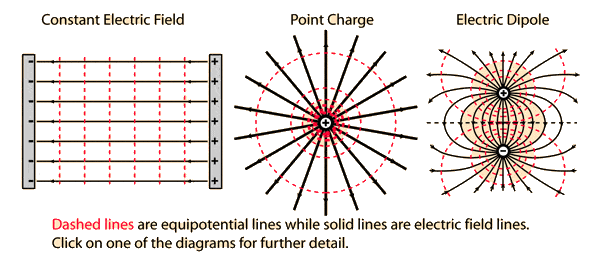•jim hardy and PhiowPhi
Thank you all!

jim hardy
Gold Member
Dearly Missed
So --- using dlgoff's rightmost picture

There'll be a minute voltage drop across any real (non-ideal) conductor.

Since current enters at lower right and exits upper left, there must be a tiny potential difference.

Draw a line made of red dashes between lower left and upper right vertices ....
Would that line represent locus of potential halfway between head and tail of your arrow?
Could your arrow represent the miniscule electric field that's moving charge ?
Would there be other electric field arrows surrounding yours, bent convex like the field lines in Don's rightmost sketch ?
Could you draw in dashed equipotential lines perpendicular to the field lines?
Could you go back to 8th grade geometry and figure out their loci? Seems to me they'd be segments of circles with centers lying on extensions of your arrow .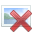it was kinda fun actually, remembering my old fluid flow class and our primitive graphical solutions . Ahhh nostalgia. 1965 was a good year.

old jim

Something that I'm reminded of from another thread, is that current is a scalar, so it makes sense to have current flow moving everywhere.
But then again, how can this diagram be true?Specifically in calculations that would require me to know where current is flowing, but then again it shouldn't have a direction because it's scalar, but then again the diagram is somewhat correct because of the potential difference intuitively making me assume that current will flow from bottom right to upper left as diagram above.

So I could say, current will flow as diagramed above because it will flow from high to low potentials and since the terminals are where they are... it should be like that.

sophiecentaur
Gold Member
Something that I'm reminded of from another thread, is that current is a scalar, so it makes sense to have current flow moving everywhere.
How can Current be a scalar? Remember Flemming's Left Hand Motor Rule? You use your finger to describe the direction of the current and that tells you the direction of the Force. If current were not a Vector quantity, you couldn't have that rule and motors wouldn't work. Just look at Ampere's Law etc. etc. ;)

Well one reason why ##I## is scalar is because it can't be added. Vectors could add up while current does not(from KCL) and there are other reasons, while as for the direction it doesn't have one, but I believe it's orientation is based on the signs in the mathematics, not so sure how to explain it well... but @vanhees71 explained it quite beautifully here, post #13.

jim hardy
Gold Member
Dearly Missed

current certainly looks and feels like a vector so long as it's constrained to a wire

but i'm told that physicists define it as integral of dot product of current density and area
and dot product gives a scalar ?

I =s J dot ds

as in this post

If anyone has a down to earth explanation it'd be you.

Perhaps as vanhees suggested our right hand rules are written for scalar current multiplied by vector tangent to the wire?

hyperphysics let me down on this one.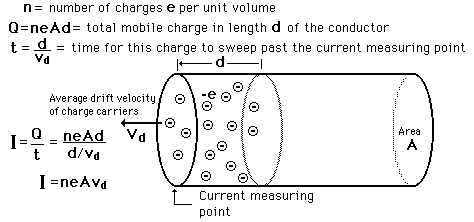no mention of vectors at all.

old jim

sophiecentaur
Gold Member
@Jim
Drift velocity does imply direction so there is an implication of vector there. Having looked at those other learned threads, I think the answer is that it is and it isn't a vector - depending upon what sort of vector you're talking about. The path of charges on a conductor of changing width can't be the same all over the csa of the conductor (obvious) and if you take a small element somewhere, the charges will be moving in a different direction from at other places. So you don't need to constrain the current along an ideal wire for a Magnitude and Direction to be associated with the current. But, as 'they' say, you can't do simple vector addition to current so it has to be a 'special' kind of vector. I guess it's all to do with Flux and Area Vectors. We could probably leave it at that - unless we both / all want to do a lot more homework on Vectors and Vector Fields so that we' know what we're talking about. (And we'd hate not to, would't we?)

vanhees71
Gold Member
2021 Award
An electric current always refers to a surface and is the amount of electric charge, ##\mathrm{d} Q##, running through the surface in a small time interval ##\mathrm{d} t## devided by ##\mathrm{d} t##. So it's a scalar quantity, because charge and time are scalars.

The electric current density is much more intuitive, because it's a vector and given by the density of charge times the velocity of the charges, ##\vec{j}=\rho \vec{v}##. Now if you have a surface ##F## you can devide it into small area elements and assign area-element vectors to them. The vector has a magnitude given by the area of the area element and is directed perpendicular to the surface. It's your choice, which of the two possible orientations you choose. Then
##I=\int_{F} \mathrm{d}^2 \vec{F} \cdot \vec{j}##
is the current as defined above. It's a scalar, because the dot product of two vectors is a scalar, and an integral can be seen as the limit of a sum, and the sum of scalars is still a scalar.

To understand the above formula, it should be clear that in a time ##\mathrm{d} t## the charges contained in the volume ##\mathrm{d} V=\mathrm{d} t \vec{v} \cdot \mathrm{d}^2 \vec{F}## are streaming through the surface, and with the density the corresponding charge contained in that volume is ##\rho \mathrm{d} V=\mathrm{d} t \rho \vec{v} \cdot \mathrm{d}^2 \vec{F}=\mathrm{d} t \vec{j} \cdot \mathrm{d} \vec{F}##.

I hope that helps to clarify the physicists' convention to name the quantities "electric current" (scalar) and "electric current density" (vector).

jim hardy
Gold Member
Dearly Missed
Thank you Sophie

Vector Calculus and i had an abusive relationship and i was the abusee.....

There are a lot of such "fine points" in my knowledge base about which i am uncertain
so i carry an awareness that i'm using a simplification of exact science

A thin wire constrains its current to vector-like behavior is my simplification for this one.

In my world , circuits, we rarely had to deal with fields.

Thanks again

old jim

sophiecentaur
Gold Member
Fields were somewhere to erect Poles in, probably.

•jim hardy
jim hardy
Gold Member
Dearly Missed
Thank you vanhees for that most excellent and eloquent explanation

i must work on my mental image of "area-element vectors" , need a picture.

and what is the d2 term in d2F ?

rest of above math is straightforward

thanks again

jim hardy
Gold Member
Dearly Missed
Fields were somewhere to erect Poles in, probably.

The course was for me Dante's fifth circle.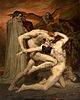Well, a lot of problems I've studied, I'm obligated to state the direction current... so bear with me here. The following diagrams are they correct in-terms of their direction?And,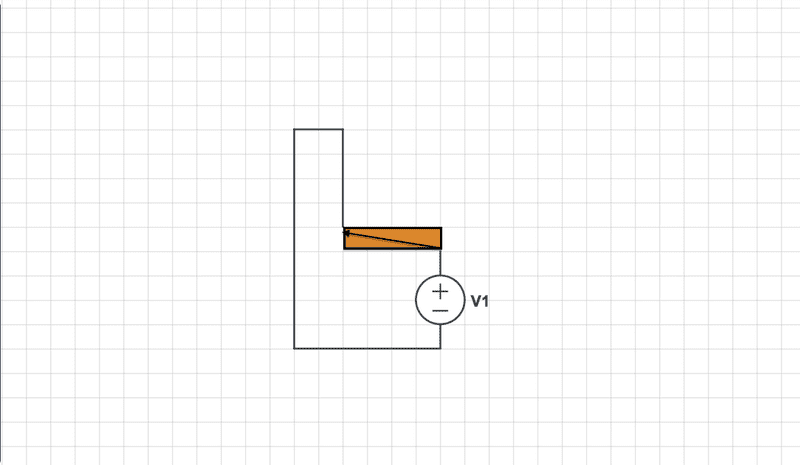I wasn't sure If I'm right or wrong here...

jim hardy
Gold Member
Dearly Missed
just be aware current isn't constrained to straight lines
recall a conversation about equipotential lines with flow perpendicular..
a diagonal line from lower left to upper right corner will be at potential halfway between other two corners

View attachment 78006

#### Attachments

sophiecentaur
•